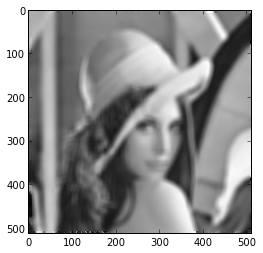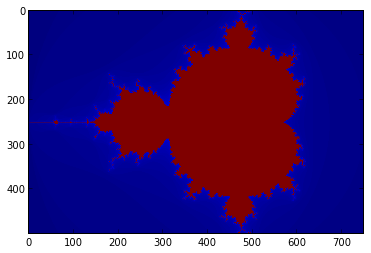This example assumes the notebook server has been called with ipython notebook --pylab inline and the trunk version of numba at Github.

In :
import numpy as np
from numba import autojit, jit, double


Numba provides two major decorators: jit and autojit.

The jit decorator returns a compiled version of the function using the input types and the output types of the function. You can specify the type using out_type(in_type, ...) syntax. Array inputs can be specified using [:,:] appended to the type.

The autojit decorator does not require you to specify any types. It watches for what types you call the function with and infers the type of the return. If there is a previously compiled version of the code available it uses it, if not it generates machine code for the function and then executes that code.

In :
def sum(arr):
M, N = arr.shape
sum = 0.0
for i in range(M):
for j in range(N):
sum += arr[i,j]
return sum
fastsum = jit('f8(f8[:,:])')(sum)
flexsum = autojit(sum)

In :
arr2d = np.arange(600,dtype=float).reshape(20,30)
print sum(arr2d)
print fastsum(arr2d)
print flexsum(arr2d)
print flexsum(arr2d.astype(int))

179700.0
179700.0
179700.0
179700.0

In :
%timeit sum(arr2d)

1000 loops, best of 3: 393 us per loop

In :
%timeit fastsum(arr2d)

100000 loops, best of 3: 2.88 us per loop

In :
393 / 2.88 # speedup

Out:
136.45833333333334
In :
%timeit arr2d.sum()

100000 loops, best of 3: 7.9 us per loop

In :
7.9 / 2.88 # even provides a speedup over general-purpose NumPy surm

Out:
2.743055555555556

The speed-up is even more pronounced the more inner loops in the code. Here is an image processing example:

In :
@jit('void(f8[:,:],f8[:,:],f8[:,:])')
def filter(image, filt, output):
M, N = image.shape
m, n = filt.shape
for i in range(m//2, M-m//2):
for j in range(n//2, N-n//2):
result = 0.0
for k in range(m):
for l in range(n):
result += image[i+k-m//2,j+l-n//2]*filt[k, l]
output[i,j] = result

from scipy.misc import lena
import time
image = lena().astype('double')
filt = np.ones((15,15),dtype='double')
filt /= filt.sum()
output = image.copy()
filter(image, filt, output)
gray()
imshow(output)
start = time.time()
filter(image[:100,:100], filt, output[:100,:100])
fast = time.time() - start
start = time.time()
filter.py_func(image[:100,:100], filt, output[:100,:100])
slow = time.time() - start
print "Python: %f s; Numba: %f ms; Speed up is %f" % (slow, fast*1000, slow / fast)

Python: 2.717911 s; Numba: 4.322052 ms; Speed up is 628.847363You can call Numba-created functions from other Numba-created functions

In :
@autojit
def mandel(x, y, max_iters):
"""
Given the real and imaginary parts of a complex number,
determine if it is a candidate for membership in the Mandelbrot
set given a fixed number of iterations.
"""
i = 0
c = complex(x, y)
z = 0.0j
for i in range(max_iters):
z = z**2 + c
if abs(z)**2 >= 4:
return i

return 255

@autojit
def create_fractal(min_x, max_x, min_y, max_y, image, iters):
height = image.shape
width = image.shape

pixel_size_x = (max_x - min_x) / width
pixel_size_y = (max_y - min_y) / height
for x in range(width):
real = min_x + x * pixel_size_x
for y in range(height):
imag = min_y + y * pixel_size_y
color = mandel(real, imag, iters)
image[y, x] = color

return image

image = np.zeros((500, 750), dtype=np.uint8)
imshow(create_fractal(-2.0, 1.0, -1.0, 1.0, image, 20))
jet()In :
%timeit create_fractal(-2.0, 1.0, -1.0, 1.0, image, 20)

1 loops, best of 3: 1.28 s per loop

In :
%timeit create_fractal.py_func(-2.0, 1.0, -1.0, 1.0, image, 20)

1 loops, best of 3: 18.9 s per loop

In :
18.9/ 1.28 # speedup of compiling outer-loop (inner-loop mandel call is still optimized)

Out:
14.765624999999998

Numba works very well for numerical calculations and infers types for variables. You can over-ride this inference by passing in a locals dictionary to the autojit decorator. Notice how the code below shows both Python object manipulation and native manipulation

In :
class MyClass(object):
def mymethod(self, arg):
return arg * 2

@autojit(locals=dict(mydouble=double)) # specify types for local variables
def call_method(obj):
print obj.mymethod("hello")   # object result
mydouble = obj.mymethod(10.2) # native double
print mydouble * 2            # native multiplication

call_method(MyClass())

hellohello
40.8


Complex support is available as well.

In :
@autojit
def complex_support(real, imag):
c = complex(real, imag)
return (c ** 2).conjugate()

c = 2.0 + 4.0j
complex_support(c.real, c.imag), (c**2).conjugate()

Out:
((-12-16j), (-12-16j))

We can even create a function that takes a structured array as input.

In :
from numba import struct, jit2
record_type = struct([('x', double), ('y', double)])
record_dtype = record_type.get_dtype()
a = np.array([(1.0, 2.0), (3.0, 4.0)], dtype=record_dtype)

@jit2(argtypes=[record_type[:]])
def pyth(data):
result = np.empty_like(data, dtype=np.float64) # return types of numpy functions are inferred
for i in range(data.shape):
result[i] = np.sqrt(data[i].x ** 2 + data[i].y ** 2)

return result

print pyth(a)

[ 2.23606798  5.        ]

In :
print pyth.signature # inspect function signature, note inferred return type

double[:] (*)(struct { double x, double y }[:])

In :
[line for line in str(pyth.lfunc).splitlines() if 'sqrt' in line] # note native math calls

Out:
['  %90 = call double @llvm.sqrt.f64(double %89)']

The roadmap for Numba includes better error-handling, support for almost all Python syntax which gets compiled to code that either uses machine instructions or else the Python library run-time, improved support for basic types, and the ability to create objects easily.

The commercial product NumbaPro includes additional features:

• ability to create ufuncs (fast-vectorize)
• ability to target the GPU via CUDA
• ability to target multiple-cores
• array-expressions (ability to write NumPy-like code that gets compiled)